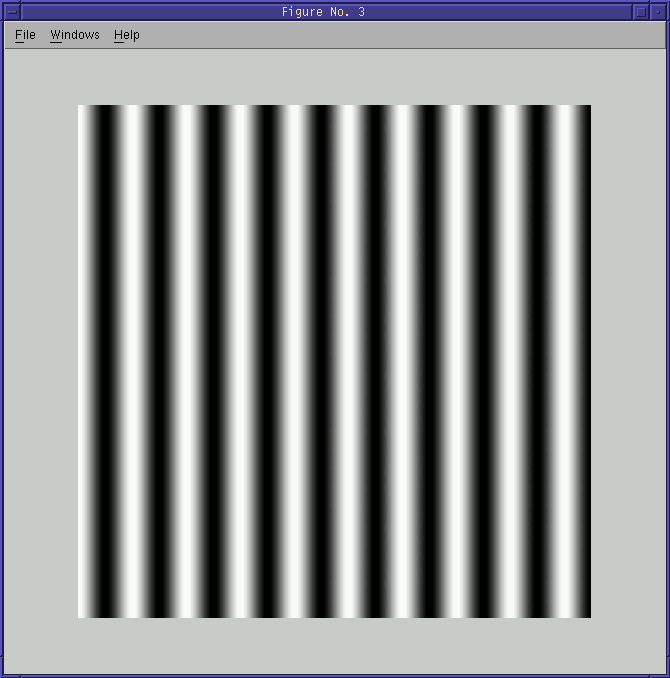## Imaging Lab 2

Ted Gould
Bryan Evenson

Code for creating Cosine image:

``` ```

``````function y = cos_im2(lambday, n)
tt=linspace(0, n, n+1);
row1 = 1 + cos(2* pi * tt/ lambday);
a1 = ones (n + 1, 1);
y = a1 * row1;
``````

First cosine wave:

``````
imshow(mat2gray(cos_im2(40, 512)));
``````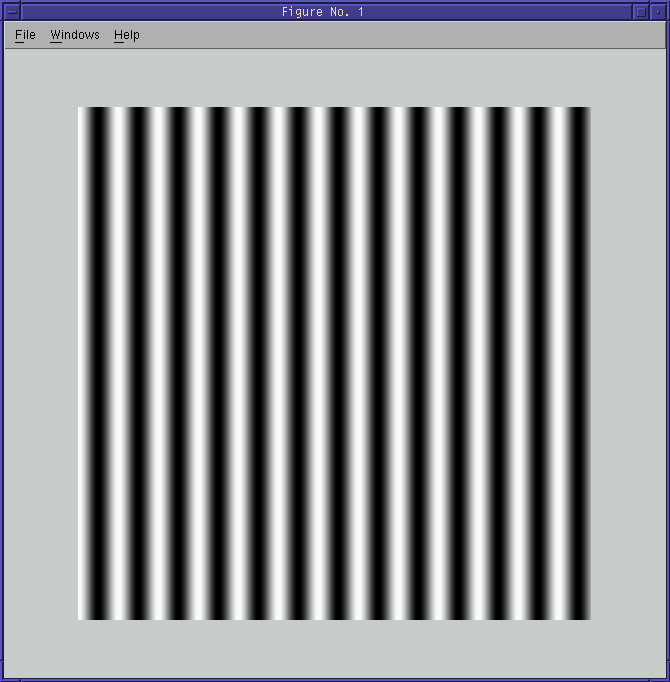FFT of First wave:

``````
imshow(mat2gray(sqrt(sqrt(abs(fftshift(fft2(cos_img2(40, 512))))))));
``````

Note: We had trouble seeing the two dots on the image. To increase the relitive intensity we took the fourth root of the entire image. We continued to do this on all of the fouier transforms throughout the lab.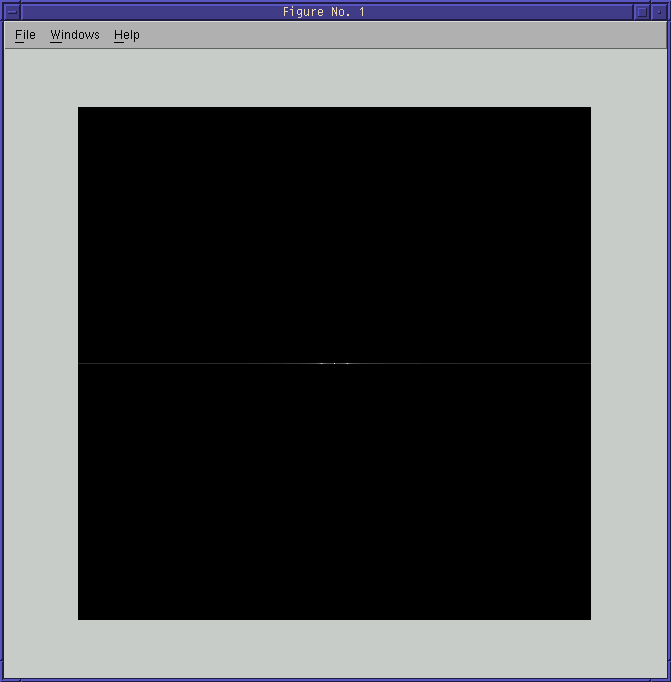Combined waves:

``````
imshow(mat2gray(cos_im2(40, 512) + cos_im2(54, 512)));
``````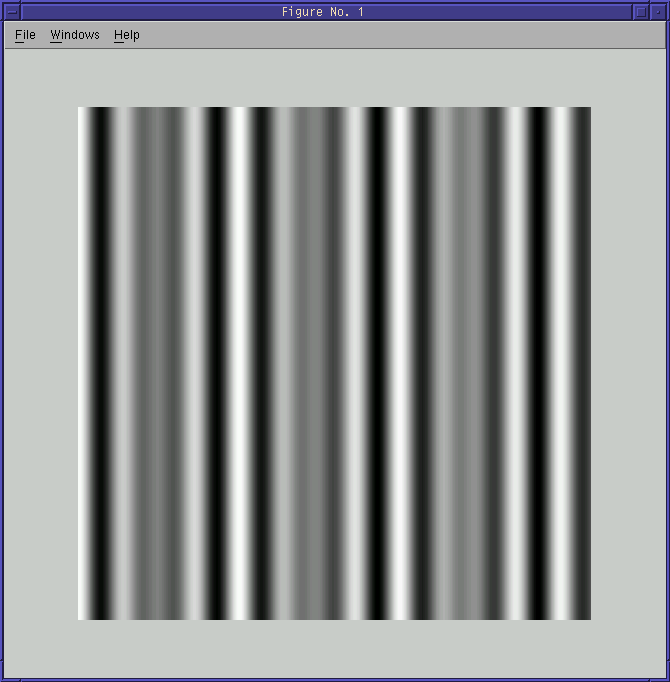Combined FFT:

``````
imshow(mat2gray(sqrt(sqrt(abs(fftshift(fft2(cos_img2(40, 512) + cos_img2(54, 512))))))));
``````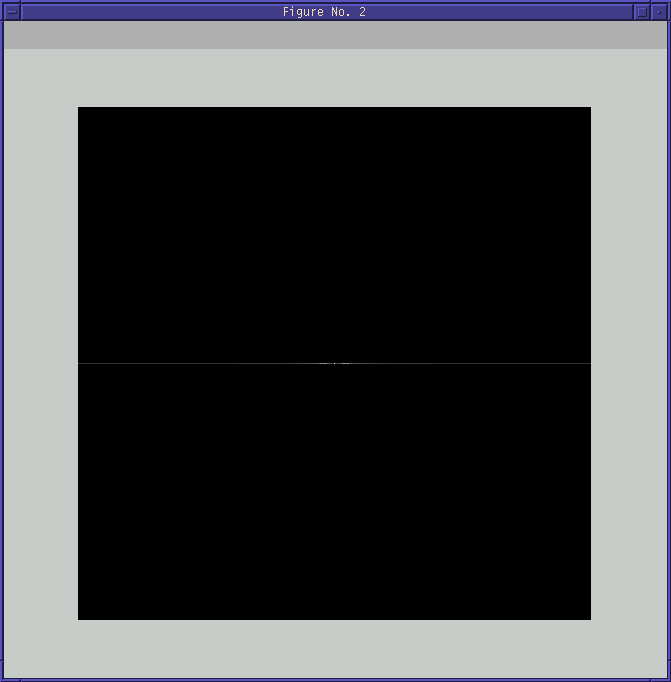Subracted Wave:

``````
X2FFT = fft2(cos_img2(40, 512) + cos_img2(54, 512));
XFFT = fft2(cos_img2(40, 512));
X3FFT = X2FFT - XFFT;
imshow(mat2gray(abs(ifft(X3FFT))));
``````